# Colors for Plotting

The difference between a simple graph and a visually stunning graph is of course a matter of many features. But one of the biggest contributors to the “wow” factors that often accompanies R graphics is the careful use of color. By default, R graphs tend to be black-and-white and, in fact, rather unattractive. But R provides many functions for carefully controlling the colors that are used in plots. This tutorial looks at some of these functions.

To start, we need to have a baseline graph. We'll use a simple scatterplot. Let's start with some x and y data vectors and a z grouping factor that we'll use later:

set.seed(100)
z <- sample(1:4, 100, TRUE)
x <- rnorm(100)
y <- rnorm(100)


Let's draw the basic scatterplot:

plot(x, y, pch = 15)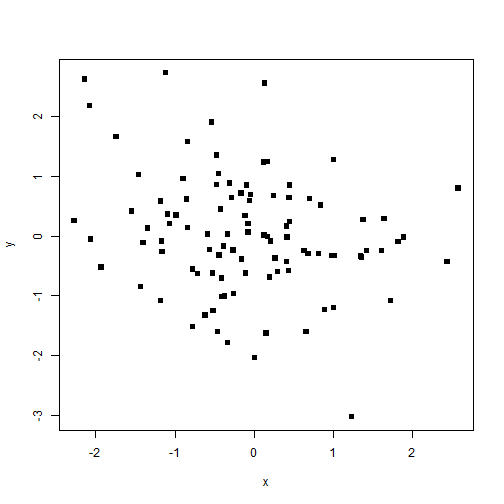By default, the points in this plot are black. But we can change that color by specifying a col argument and a character string containing a color. For example, we could make the points red:

plot(x, y, pch = 15, col = "red")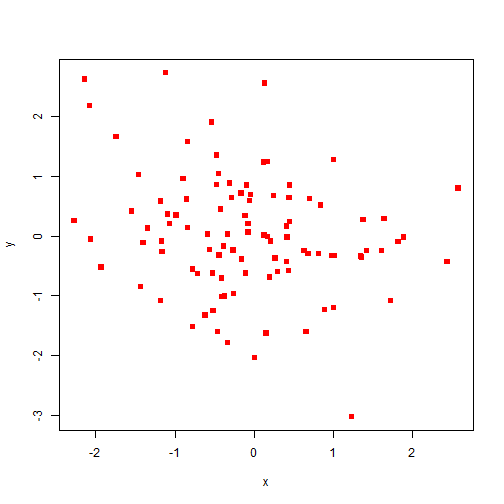or blue:

plot(x, y, pch = 15, col = "blue")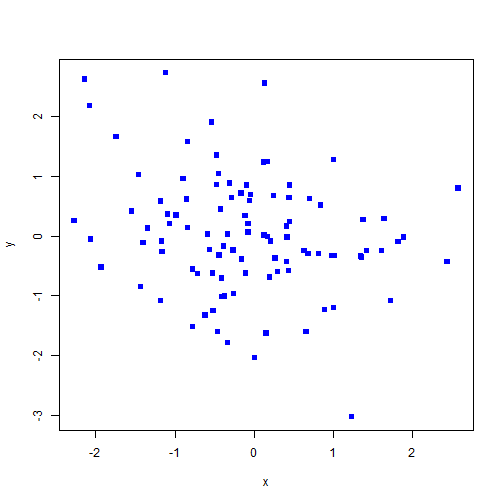R comes with hundreds of colors, which we can see using the colors() function. Let's see the first 25 colors in this:

colors()[1:25]

##   "white"          "aliceblue"      "antiquewhite"   "antiquewhite1"
##   "antiquewhite2"  "antiquewhite3"  "antiquewhite4"  "aquamarine"
##   "aquamarine1"    "aquamarine2"    "aquamarine3"    "aquamarine4"
##  "azure"          "azure1"         "azure2"         "azure3"
##  "azure4"         "beige"          "bisque"         "bisque1"
##  "bisque2"        "bisque3"        "bisque4"        "black"
##  "blanchedalmond"


You can specify any of these colors as is.

## Color Vector Recycling

An important aspect of R's use of the col argument is the notion of vector recyling. R expects the col argument to have the same length as the number of things its plotting (in this case the number of points). So when we specify col='red', R actually “recycles” the color red for each point, effectively constructing a vector like c('red','red','red',...) equal to the length of our data. We can take advantage of recycling to specify multiple colors. For example, we can specify every other point in our data as being red and blue:

plot(x, y, pch = 15, col = c("red", "blue"))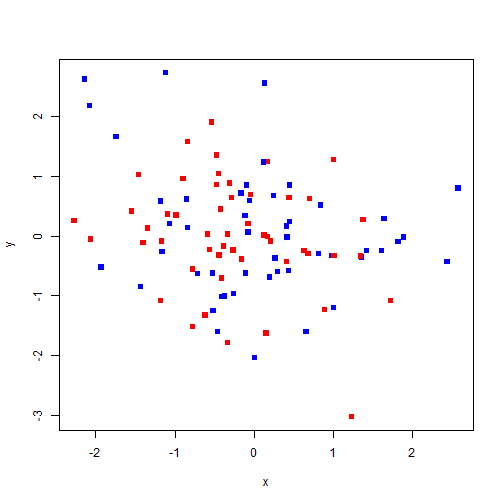Of course, these colors are not substantively meaningful. Our data are not organized in an alternating fashion. We did, however, have a grouping factor z that takes four levels. We can imagine that these are four substantively important groups in our data that we would like to highlight with different colors. To do that, we could specify a vector of four colors and index it using our z vector:

plot(x, y, pch = 15, col = c("red", "blue", "green", "orange")[z])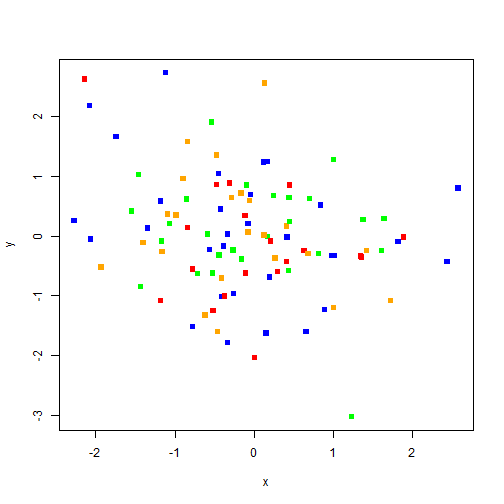Now, the four groups each have their own color in the resulting plot. Another strategy is to use the pch (“point character”) argument to identify groups, which we can do using the same logic:

plot(x, y, pch = c(15, 16, 17, 18)[z])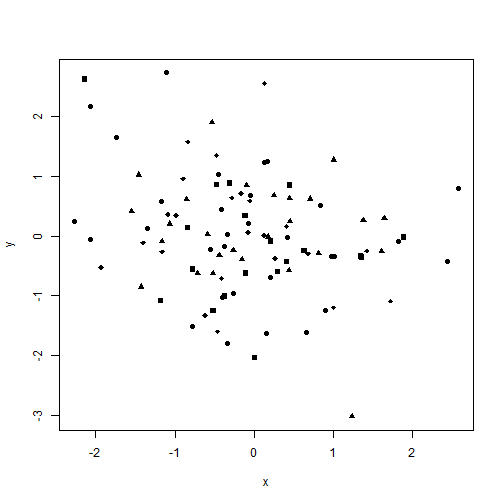But I think colors look better here than different shapes. Of course, sometimes we have to print in grayscale or monochrome, so finding the best combination of shapes and colors may take a bit of work.

## Color generation functions

In addition to the named colors, R can also generate any other color pattern in the rainbow using one of several functions. For example, the rgb function can generate a color based on levels of Red, Green, and Blue (thus the rgb name). For example, the color red is simply:

rgb(1, 0, 0)

##  "#FF0000"


The result is the color red expressed in hexidecimal format. Two other functions - hsv and hcl - let you specify colors in other ways, but rgb is the easiest, in part, because hexidecimal format is widely used in web publishing so there are many tools online for figuring out how to create the color you want as a combination of red, green, and blue. We can see that specifying col='red' or col=rgb(1,0,0) produce the same graphical result:

plot(x, y, pch = 15, col = "red")plot(x, y, pch = 15, col = rgb(1, 0, 0))But rgb (and the other color-generation functions) are also “vectorized”, meaning that we can supply them with a vector of numbers in order to obtain different shades. For example, to get four shades of red, we can type:

rgb((1:4)/4, 0, 0)

##  "#400000" "#800000" "#BF0000" "#FF0000"


If we index this with z (as we did above), we get a plot where are different groups are represented by different shades of red:

plot(x, y, pch = 15, col = rgb((1:4)/4, 0, 0)[z])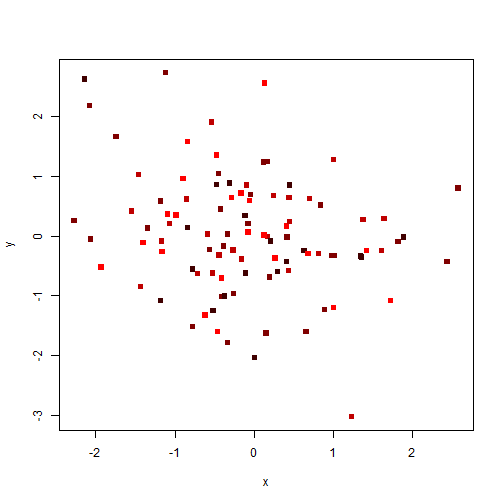When we have to print in grayscale, R also supplies a function for building shades of gray, which is called - unsurprisingly - gray. The gray function takes a number between 0 and 1 that specifies a shade of gray between black (0) and white (1):

gray(0.5)

##  "#808080"


The response is, again, a hexidecimal color representation. Like rgb, gray is vectorized and we can use it to color our plot:

gray((1:4)/6)

##  "#2B2B2B" "#555555" "#808080" "#AAAAAA"

plot(x, y, pch = 15, col = gray((1:4)/6)[z])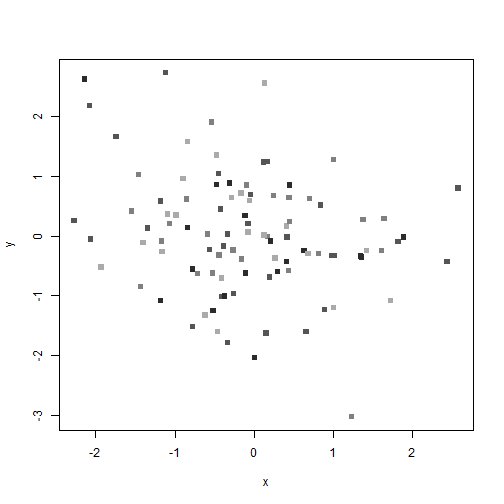But R doesn't restrict us to one color palette - just one color or just grayscale. We can also produce “rainbows” of color. For example, we could use the rainbow function to get a rainbow of four different colors and use it on our plot.

plot(x, y, pch = 15, col = rainbow(4)[z])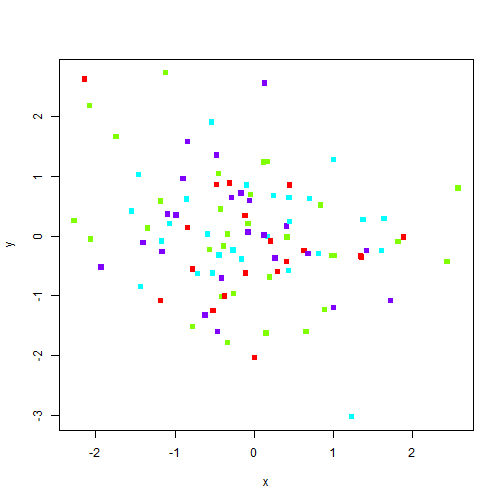rainbow takes additional arguments, such as start and end that specify where on the rainbow (as measured from 0 to 1) the colors should come from. So, specifying low values for start and end will make a red/yellow-ish plot, middling values will produce a green/blue-ish plot, and high values will prdocue a blue/purple-ish plot:

plot(x, y, pch = 15, col = rainbow(4, start = 0, end = 0.25)[z])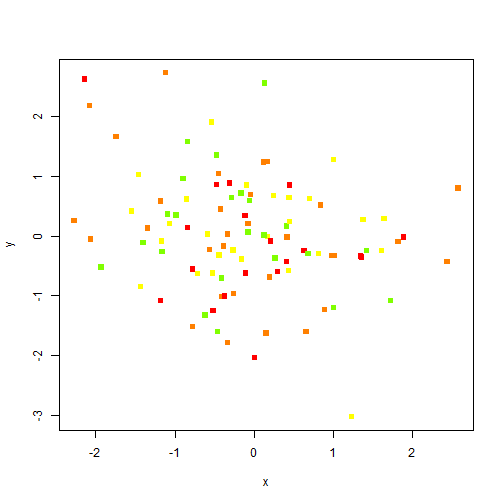plot(x, y, pch = 15, col = rainbow(4, start = 0.35, end = 0.6)[z])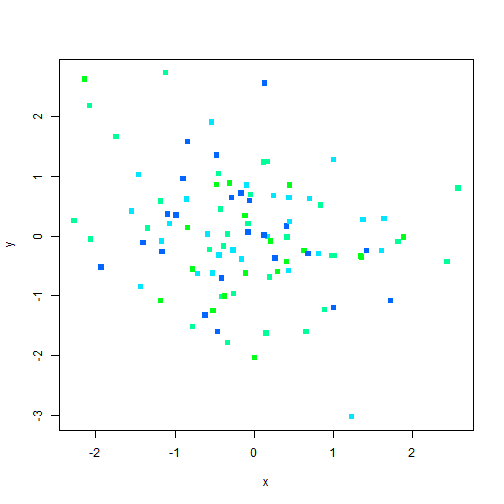plot(x, y, pch = 15, col = rainbow(4, start = 0.7, end = 0.9)[z])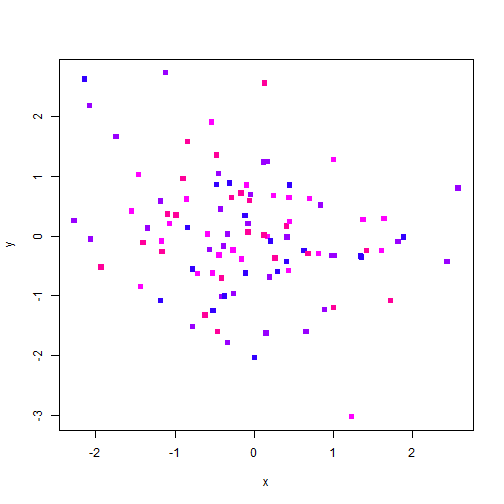## Color as data ##

Above we've used color to convey groups within the data. But we can also use color to convey a third variable on our two-dimensional plot. For example, we can imagine that we have some outcome val to which x and y each contribute. We want to see the level of val as it is affected by both x and y. Let's start by creating the val vector as a function of x and y and then use it as a color value:

val <- x + y


Then let's rescale val to be between 0 and 1 to make it easier to use in our color functions:

valcol <- (val + abs(min(val)))/max(val + abs(min(val)))


Now we can use the valcol vector to color our plot using gray:

plot(x, y, pch = 15, col = gray(valcol))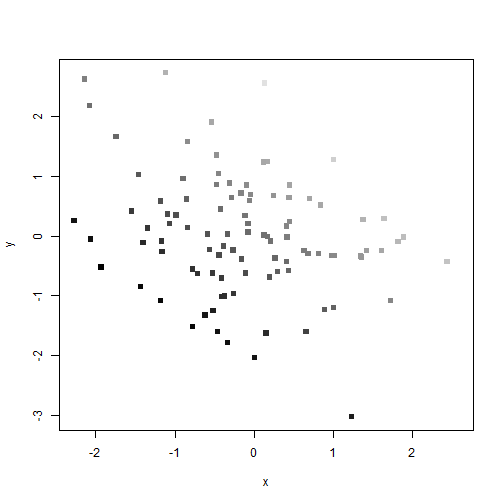We could also use rgb to create a spectrum of blues:

plot(x, y, pch = 15, col = rgb(0, 0, valcol))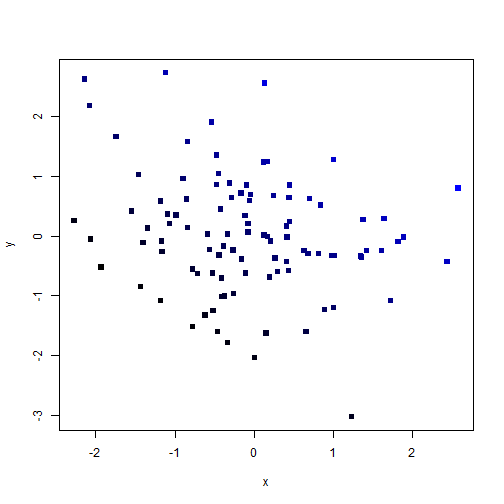There are endless other options, but this conveys the basic principles of plot coloring which rely on named colors or a color generation function, and the general R principles of recycling and vectorization.